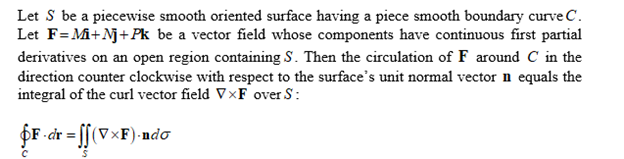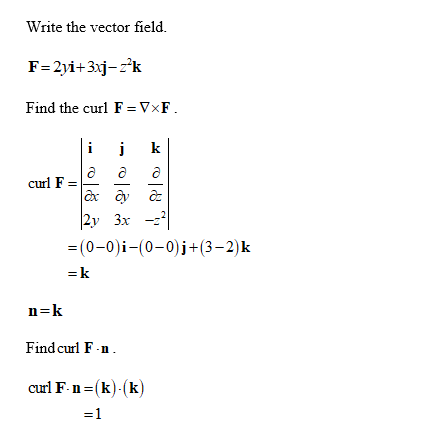# use the surface integral in Stokes’ Theorem to calculate the circulation of the field F around the curve C in the indicated direction.F = 2yi + 3xj - z2kC: The circle x2 + y2 = 9 in the xy-plane, counterclockwise when viewed from above.

Question
2 views

use the surface integral in Stokes’ Theorem to calculate the circulation of the field F around the curve C in the indicated direction.

F = 2yi + 3xj - z2k

C: The circle x2 + y2 = 9 in the xy-plane, counterclockwise when viewed from above.

check_circle

Step 1

Stokes’ Theorem:Step 2...

### Want to see the full answer?

See Solution

#### Want to see this answer and more?

Solutions are written by subject experts who are available 24/7. Questions are typically answered within 1 hour.*

See Solution
*Response times may vary by subject and question.
Tagged in